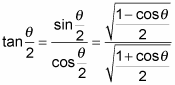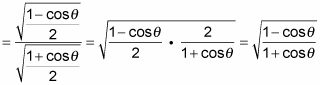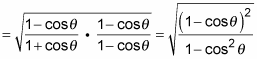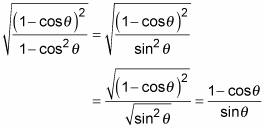##### Trigonometry For DummiesThe half-angle trig identity for tangent has two versions. Rather than this being a nuisance, having more than one option is really rather nice, because you can choose the version that works best for your situation. The half-angle formulas for the tangent involve both sine and cosine, but those functions switch places in the numerator and denominator of the fraction.

Sometimes the sine of a function doesn’t have a radical in its exact value when the cosine does (or vice versa). Depending on the sine and cosine values, you choose the version of the half-angle tangent identity that’ll be easiest to work with after you input the values. The math is easier when you don’t have to worry about those radicals in the denominator.

First, where do these half-angle tangent identities come from?

1. Use the ratio identity for tangent and fill in the half-angle identities for sine and cosine.You can leave off the ± sign because you won’t have to choose which sign to use with the tangent identity — the square-root sign squares out of the equation.

2. Put the numerator and denominator under the same radical and then simplify the complex fraction.3. Multiply the numerator and denominator by the conjugate (same terms, different sign) of the denominator.4. Replace the denominator by using the Pythagorean identity and then simplify by putting the radical over the numerator and denominator, individually.To find the other form of this half-angle tangent identity, change Step 3 by multiplying the numerator and denominator of the fraction by the conjugate of the numerator instead of the denominator.